# DENSITY_MODEL

Specifies a density model.

AcuSolve Command

## Syntax

DENSITY_MODEL("name") {parameters...}

User-given name.

## Parameters

type (enumerated) [=none]
Type of the density model.
constant or const
Constant density. Requires density.
boussinesq
Boussinesq approximation. Requires density, expansivity_type and reference_temperature.
isentropic
Isentropic gas. Requires density, specific_heat_ratio and reference_pressure, and also absolute_pressure_offset from the EQUATION command.
ideal_gas
Ideal gas law. Requires gas_constant, and also absolute_pressure_offset and absolute_temperature_offset from the EQUATION command.
piecewise_linear or linear
Piecewise linear curve fit. Requires curve_fit_values and curve_fit_variable.
cubic_spline or spline
Cubic spline curve fit. Requires curve_fit_values and curve_fit_variable.
user_function or user
User-defined function. Requires user_function, user_values and user_strings.
density or dens (real) >0 [=1]
Constant value of the density when used with constant and boussinesq types, and value of the reference density when used with isentropic type.
expansivity_type or expans_type (enumerated) [=constant]
Type of the expansivity function used for the Boussinesq approximation. Used with boussinesq type.
constant or const
Constant expansivity. Requires expansivity.
expansivity or expans (real) >=0 [=1]
Constant value of the expansivity. Used with constant expansivity type.
reference_temperature or ref_temp (real) [=273.15]
Reference temperature of the Boussinesq approximation. Used with boussinesq type.
reference_pressure or ref_pres (real) [=0]
Value of the reference pressure. Used with isentropic type.
specific_heat_ratio (real) >=1 [=1.4]
Value of the specific heat ratio. Used with isentropic type.
gas_constant (real) [=287.058]
Value of the gas constant. Used with ideal_gas type.
isothermal_compressibility (real) >=0 [=0]
Value of the isothermal compressibility.
curve_fit_values or curve_values (array) [={0,0}]
A two-column array of independent-variable/density data values. Used with piecewise_linear and cubic_spline types.
curve_fit_variable or curve_var (enumerated) [=temperature]
Independent variable of the curve fit. Used with piecewise_linear and cubic_spline types.
x_coordinate or xcrd
X-component of coordinates.
y_coordinate or ycrd
Y-component of coordinates.
z_coordinate or zcrd
Z-component of coordinates.
x_reference_coordinate or xrefcrd
X-component of reference coordinates.
y_reference_coordinate or yrefcrd
Y-component of reference coordinates.
z_reference_coordinate or zrefcrd
Z-component of reference coordinates.
pressure or pres
Pressure.
temperature or temp
Temperature.
species_1 or spec1
Species 1.
species_2 or spec2
Species 2.
species_3 or spec3
Species 3.
species_4 or spec4
Species 4.
species_5 or spec5
Species 5.
species_6 or spec6
Species 6.
species_7 or spec7
Species 7.
species_8 or spec8
Species 8.
species_9 or spec9
Species 9.
user_function or user (string) [no default]
Name of the user-defined function. Used with user_function type.
user_values (array) [={}]
Array of values to be passed to the user-defined function. Used with user_function type.
user_strings (list) [={}]
Array of strings to be passed to the user-defined function. Used with user_function type.

## Description

This command specifies a density model for element sets. All types of element sets, fluid, solid, and shell, require a density model.

DENSITY_MODEL commands are referenced by MATERIAL_MODEL commands, which in turn are referenced by ELEMENT_SET commands:
DENSITY_MODEL( "my density model" ) {
type                                = constant
density                             = 1.225
isothermal_compressibility          = 0
}
MATERIAL_MODEL( "my material model" ) {
density_model                       = "my density model"
...
}
ELEMENT_SET( "fluid elements" ) {
material_model                      = "my material model"
...
}

A constant density model uses a constant density for the entire element set, as in the above example.

A density model of type boussinesq employs the Boussinesq approximation. The variation in density is assumed to be a linear function of temperature and to be relatively small. Hence, as a first level approximation, the variation of density is ignored for all terms except the body force term. The effect of the density variation on the body force is modeled by scaling the body force resulting from the GRAVITY, ROTATION_FORCE, and REFERENCE_FRAME commands by: (1)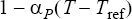where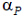is the thermal expansivity, given by expansivity; T is the temperature; and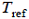is the temperature at which the density equals the given constant density value, given by reference_temperature. For example,
DENSITY_MODEL( "air" ) {
type                      = boussinesq
density                   = 1.225
expansivity_type          = constant
expansivity               = 0.0034722
reference_temperature     = 288
}

The Boussinesq model assumes that the problem contains a temperature field; see the EQUATION command. If the problem does not contain any temperature equation, then a boussinesq density model is internally converted to a constant density model.

An isentropic (constant entropy) gas may be modeled with an isentropic type. This is a full variable-density model where the variation in density is taken into account in all terms. For example,
DENSITY_MODEL( "isentropic air" ) {
type                    = isentropic
density                 = 1.225
specific_heat_ratio     = 1.4
reference_pressure      = 0
}
The density is given by: (2)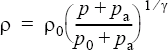where $\rho$ is the density, given by this command; $\rho$ o is the reference density, given by density; p is the pressure; po is the reference pressure, given by reference_pressure; $\gamma$ is the specific heat ratio, given by specific_heat_ratio; and pa is the absolute pressure offset, given by absolute_pressure_offset from the EQUATION command.

The specific heat ratio for the ideal gas law is computed internally through Mayer’s relation by (3)
$\gamma =\frac{{C}_{p}\left(T\right)}{\left({C}_{p}\left(T\right)-R\right)}$

where ${C}_{p}$ is the specific heat capacity; T is the temperature; R is the gas constant.

A density model based on the ideal gas law is provided by the ideal_gas type. This is a full variable-density model where the variation in density is taken into account in all terms. For example,
DENSITY_MODEL( "ideal gas air" ) {
type              = ideal_gas
gas_constant      = 287.058
}
The density is given by:(4)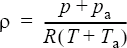where $\rho$ is the density, given by this command; R is the gas constant, given by gas_constant; p is the pressure; T is the temperature; and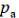and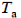are the absolute pressure and temperature offsets, given by absolute_pressure_offset and absolute_temperature_offset from the EQUATION command. This model requires the availability of the temperature field; an error will be issued otherwise. When modeling compressible flow the use of absolute_pressure_offset and absolute_temperature_offset is not recommended. Instead, you should define boundary and initial conditions in terms of absolute values.

Pseudo compressibility may be modeled by adding the isothermal compressibility term to the continuity equation:(5)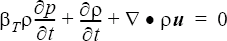where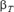is the isothermal compressibility, given by isothermal_compressibility; $\rho$ is density; p is pressure; t is time; and u is the velocity vector. Isothermal compressibility is defined by:(6)Pseudo compressibility is often used along with constant type to model wind-generated noise. Pseudo compressibility should not be activated for flow = compressible_navier_stokes.

Full variable-density models are also supported by the piecewise_linear, cubic_spline, and user_function types. As opposed to the Boussinesq type, the density for these types is variable in all terms.

Density models of types piecewise_linear and cubic_spline may be used to define density as a function of a single independent variable. For example,
DENSITY_MODEL( "air" ) {
type                      = piecewise_linear
curve_fit_values          = { 60, 1.05943 ;
160, 0.814836 ;
260, 0.662024 ;
360, 0.557587 ; }
curve_fit_variable      = temperature
}

gives a density model by defining density as a function of temperature. Use caution when defining density as a function of pressure; this can easily lead to instability.

The curve_fit_values is a two-column array corresponding to the independent variable, temperature, in this case, and density. The independent variable values must be in ascending order. The limit point values of the curve fit are used when curve_fit_variable falls outside of the curve fit limits.

The curve_fit_values data may be read from a file. For the above example, the curve fit values may be placed in a file, say density.mks:
60      1.05943
160     0.814836
260     0.662024
360     0.557587
DENSITY_MODEL( "air" ) {
type                   = piecewise_linear
curve_fit_values       = Read ( "density.mks" )
curve_fit_variable     = temperature
}

A density of type user_function may be used to model more complex behaviors; see the AcuSolve User-Defined Functions Manual for a detailed description of user-defined functions.

For example, consider a version of the Boussinesq density model discussed above. Here, the density variation appears in all terms but you "clip" the density to that corresponding to 10 degrees above and below the reference temperature. The derivative of density with respect to temperature is also returned; this Jacobian is needed to form the appropriate contribution to the left-hand side matrix. If it is not returned, no error is issued but the Jacobian is assumed to be zero and nonlinear convergence may suffer.
Note: Only Jacobians with respect to temperature are supported currently.
The density may also be a function of pressure and species, but extreme caution must be taken since Jacobians with respect to these variables are not supported. The input command may be given by:
DENSITY_MODEL( "thermal air" ) {
type                 = user_function
user_function        = "usrDensity"
user_values          = { 1.225,         # reference density
288,         # reference temperature
0.0034722 }    # expansivity
}
where the user-defined function "usrDensity" may be implemented as follows:
#include "acusim.h"
#include "udf.h"
UDF_PROTOTYPE( usrDensity ) ;                                        /* function prototype               */
Void usrDensity (
UdfHd                 udfHd,                                     /* Opaque handle for accessing data */
Real*                 outVec,                                    /* Output vector                    */
Integer               nItems,                                    /* Number of elements               */
Integer               vecDim                                     /* = 1                              */
) {
Real                  expn ;                                     /* expansivity                      */
Real                  rho0 ;                                     /* reference density                */
Real                  rhoMin ;                                   /* minimum density                  */
Real                  rhoMax ;                                   /* maximum density                  */
Real                  temp0 ;                                    /* reference temperature            */
Integer               i ;                                        /* a running index                  */
Real*                 densJacTemp ;                              /* partial dens / partial temp      */
Real*                 temp ;                                     /* temperature                      */
Real*                 usrVals ;                                  /* user supplied values             */

udfCheckNumUsrVals( udfHd, 3 ) ;                                     /* check for error                  */
usrVals               = udfGetUsrVals( udfHd ) ;
rho0                  = usrVals ;
temp0                 = usrVals ;
expn                  = usrVals ;
rhoMin                = rho0 * (1 - 10 * expn ) ;
rhoMax                = rho0 * (1 + 10 * expn ) ;
temp                  = udfGetElmData( udfHd, UDF_ELM_TEMPERATURE ) ;
densJacTemp           = udfGetElmJac( udfHd, UDF_ELM_JAC_TEMPERATURE )
for ( i = 0 ; i < nItems ; i++ ) {
densJacTemp[i]     = -rho0 * expn ;
outVec[i]          = rho0 * ( 1 + expn * (temp0 - temp[i]) ) ;
if ( outVec[i] < rhoMin ) {
densJacTemp[i] = 0 ;
outVec[i]      = rhoMin ;
if ( outVec[i] > rhoMax ) {
densJacTemp[i] = 0 ;
outVec[i]      = rhoMax ;
}
}                                                                    /* end of usrDensity()              */

The dimensions of the returned density vector, outVec, and the Jacobian vector, densJacTemp, are the number of elements.